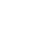Wholesaler of non-lethal self-defense devices so you can provide protection for your customers and their homes.

# How To Figure Percentage Mark Up CorrectlyHow to calculate a percentage markup from your cost is usually done wrong.

Most people do it this way…

If they want to mark something up 40%, most will multiply their cost by 1.40. Or, multiply their cost by 40% then add that number to their cost.

Same thing.

So, if they pay \$5.00 for a product, they multiply \$5.00 X 1.4 which would give me \$7.00.

Ok, if they sell the product for \$7.00, they would make a 40% profit.

WRONG!!!

To prove this, multiply \$7.00 X 40%. You get \$2.80. Subtract that from \$7.00 and you should get your cost of \$5.00. But, you don’t. You get \$4.20 which is below your cost.

However, there is a simple formula for doing this the right way.

Let’s say your cost for a product is \$5.00. You want to sell it at a 30% markup. You subtract the .30 from 1.00 and get .70.

You then divide the \$5.00 by .70 and get \$7.15. You can test this by subtracting 30% from \$7.15 which is \$2.15 and you get \$5.00.

\$7.15 X 30% = \$2.15 then \$7.15 – \$2.15 = \$5.00 or simpler \$7.15 X .70 = \$5.00

I know that’s a lot of numbers and I don’t want to confuse you, so use the formulas below to make it easier to compute percentage markup.

These are used with the starting costs.

For a 15% profit, divide the cost price by 0.85
For a 20% profit, divide the cost price by 0.8
For a 25% profit, divide the cost price by 0.75
For a 30% profit, divide the cost price by 0.7
For a 40% profit, divide the cost price by 0.6
For a 50% profit, divide the cost price by 0.5

### 3 Responses

1.steve Rodriguez says:

You also have to add in the initial shipping cost for the product.
Example you purchased 10 items from ST shipping was \$10.00. Cost per item is \$1.00.
So, your \$7.15 item is now \$8.15. If you want your 30% profit from that \$5.00 item.

1.admin says:

The cost in the example included the shipping cost. Shipping should be included in the cost, not added after you figure your markup.

2.Nick says:

Thanks Michael